# NCERT Solutions for Class 10 Maths Chapter 6 Triangles Ex 6.5

Get Free NCERT Solutions for Class 10 Maths Chapter 6 Ex 6.5 PDF. Triangles Class 10 Maths NCERT Solutions are extremely helpful while doing your homework. Exercise 6.5 Class 10 Maths NCERT Solutions were prepared by Experienced ncert-books.inTeachers. Detailed answers of all the questions in Chapter 6 Maths Class 10 Triangles Exercise 6.5 provided in NCERT TextBook.

## NCERT Solutions for Class 10 Maths Chapter 6 Triangles Ex 6.5

NCERT Solutions for Class 10 Maths Chapter 6 Triangles Ex Ex 6.5 are part of NCERT Solutions for Class 10 Maths. Here we have given NCERT Solutions for Class 10 Maths Chapter 6 Triangles Exercise 6.5

Question 1.
Sides of triangles are given below. Determine which of them are right triangles. In case of a right triangle, write the length of its hypotenuse.
(i) 7 cm, 24 cm, 25 cm
(ii) 3 cm, 8 cm, 6 cm
(iii) 50 cm, 80 cm, 100 cm
(iv) 13 cm, 12 cm, 5 cm
Solution:Question 2.
PQR is a triangle right angled at P and M is a point on QR such that PM ⊥ QR. Show that PM2 = QM X MR.
Solution:Question 3.
In the given figure, ABD is a triangle right angled at A and AC i. BD. Show that
(i) AB2 = BC.BD
(ii) AC2 = BC.DCSolution: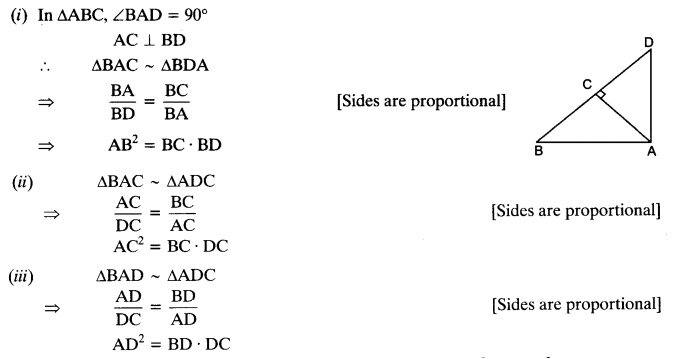Question 4.
ABC is an isosceles triangle right angled at C. Prove that AB2 = 2AC2.
Solution:Question 5.
ABC is an isosceles triangle with AC = BC. If AB2 = 2AC2 , Prove that ABC is a right triangle.
Solution:Question 6.
ABC is an equilateral triangle of side la. Find each of its altitudes.
Solution:Question 7.
Prove that the sum of the squares of the sides of a rhombus is equal to the sum of the squares of its diagonals.
Solution:Question 8.
In the given figure, O is a point in the interior of a triangle ABC, OD ⊥ BC, OE ⊥ AC and OF ⊥ AB. Show that
(i) OA2 + OB2 + OC2 – OD2 – OE2 – OF2 = AF2 + BD2 + CE2
(ii) AF2 + BD2 + CE2 = AE2 + CD2 + BF2.Solution:Question 9.
A ladder 10 m long reaches a window 8 m above the ground. ind the distance of the foot of the ladder from base of the wall.
Solution:Question 10.
A guy wire attached to a vertical pole of height 18 m is 24 m long and has a stake attached to the other end. How far from the base of the pole should the stake be driven so that the wire will be taut?
Solution: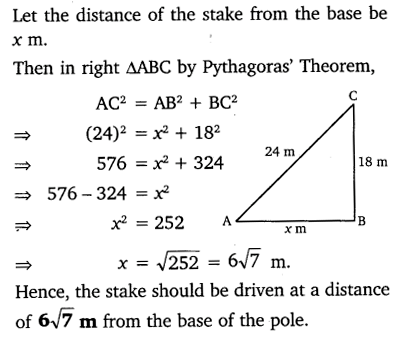Question 11.
An aeroplane leaves an airport and flies due north at a speed of 1000 km per hour. At the same time, another aeroplane leaves the same airport and flies due west at a speed of 1200 km per hour. How far apart will be the two planes after 1$\frac { 1 }{ 2 }$hours?
Solution:Question 12.
Two poles of heights 6 m and 11m stand on a plane ground. If the distance between the feet of the poles is 12 m, find the distance between their tops.
Solution:Question 13.
D and E are points on the sides CA and CB respectively of a triangle ABC right angled at C. Prove that AE2 + BD2 = AB2 + DE2.
Solution:Question 14.
The perpendicular from A on side BC of a ∆ABC intersects BC at D such that DB = 3CD (see the figure). Prove that 2AB2 = 2AC2 + BC2.Solution: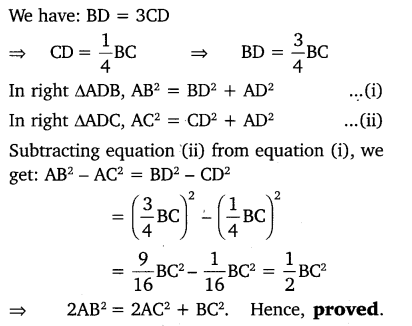Question 15.
In an equilateral triangle ABC, D is a point on side BC, such that BD =$\frac { 1 }{ 3 }$BC. Prove that 9AD2 = 7AB2.
Solution: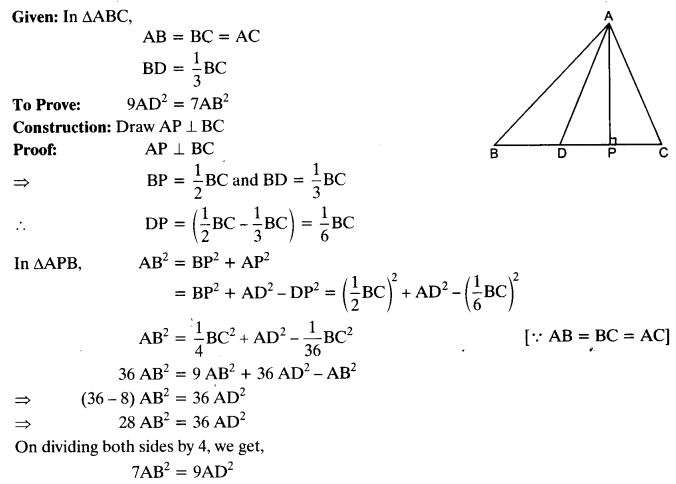Question 16.
In an equilateral triangle, prove that three times the square of one side is equal to four times the square of one of its altitudes.
Solution: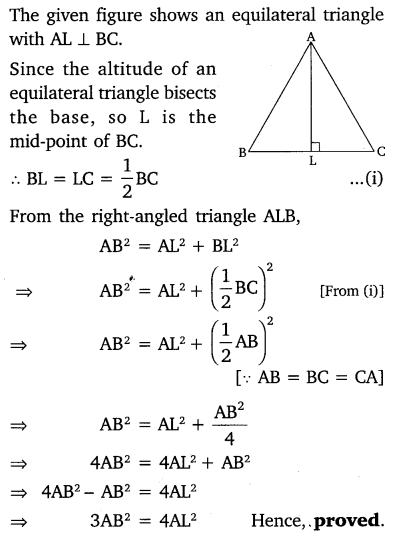Question 17.
Tick the correct answer and justify : In ∆ABC, AB = 6$\sqrt { 3 }$cm, AC = 12 cm and BC = 6 cm. The angle B is:
(a) 120°
(b) 60°
(c) 90°
(d) 45
Solution: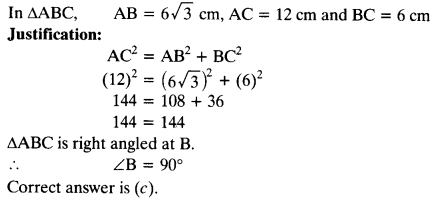error: Content is protected !!
+